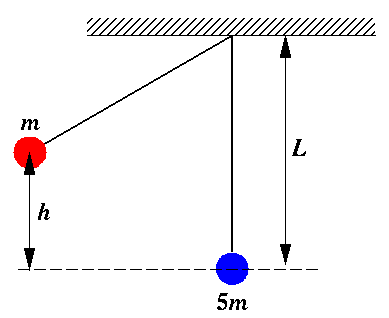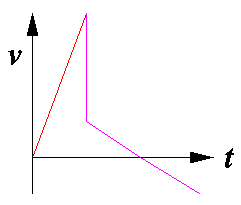Directions: Select the best possible answer from the choices A-D. Guessing will not (on average) reduce your score, but if you have no idea how to work a problem, leave it blank and I'll give you the expected ¼ point.

This is a timed test. Your start time is: 18:34

## Ballistic PendulumA red sphere (of mass m) and a blue sphere (of mass 5m) are attached to the ceiling by massless strings of identical length forming twin pendulums of length L. The red sphere is drawn to the left so that its center of mass has been raised a distance h and is then released. As the red sphere moves back through equilibrium it hits and adheres to the blue sphere. The combined system swings to the right, eventually reaching some maximum height before returning to equilibrium.

1. If the post-collision speed of the combined spheres is initially v, what maximum height will be achieved?
1. v2/(2gL)½
2. mgh
3. v2/g
4. v2/(2g)
```A B C D
```

2. Which graph best represents the horizontal velocity of the red sphere as a function of time?

ABCD```A B C D
```

The next random number would have been: .63904